Pull Down & Release to Refresh

# The Unit Circle and Trigonometry

## The Unit Circle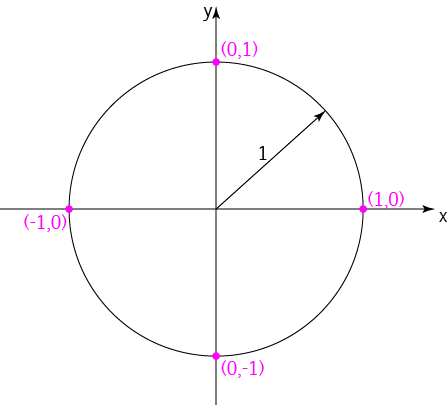The unit circle is a circle with radius $1$. We centre the unit circle at the origin ($(0,0)$) so that we can use it to talk about lengths and angles.

## Sines, Cosines and Tangents, and the Unit Circle

For the past few months, I have been teaching some American students who are preparing for their college entrance exams. They seem to base their knowledge of trigonometry on the unit circle. I haven't seen trigonometry taught this way in Australia, but the approach does point out some interesting connections between the unit circle and trigonometric functions. It's well worth having a look at.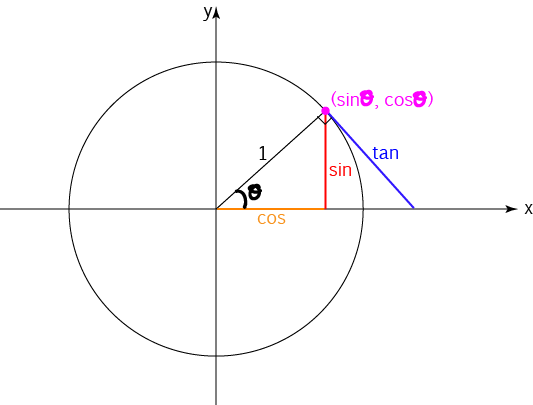We can draw a line from the centre of the unit circle to each point $(x,y)$ on its circumference. If $\theta$ is the angle that the line makes with the positive $x$-axis, then the $x$-coordinate of point $(x,y)$ is equal to $\cos \theta$, and the $y$-coordinate is equal to $\sin \theta$. The tangent of angle $\theta$ is equal to $\tan \theta = \dfrac{y}{x}$. So, we can read off these trigonometric ratios from the perpendicular distances between the point $(x,y)$ and the axes.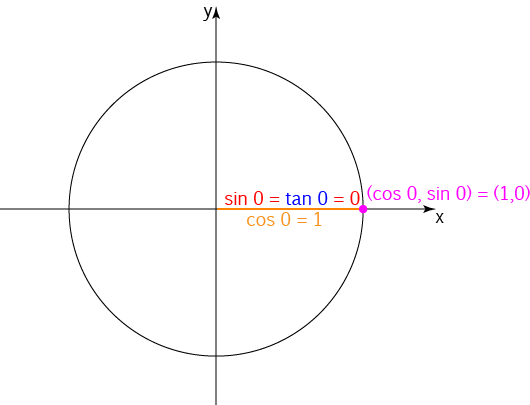If the angle $\theta$ that the line from $(0,0)$ to $(x,y)$ makes with the positive $x$-axis is $0^\circ$, then the $x$-coordinate of point $(x,y)$ is equal to $\cos 0 = 1$, and the $y$-coordinate is equal to $\sin 0 = 0$. The tangent of $0^\circ$ is equal to $\tan 0 = 0$. So, the point has coordinates $(1,0)$.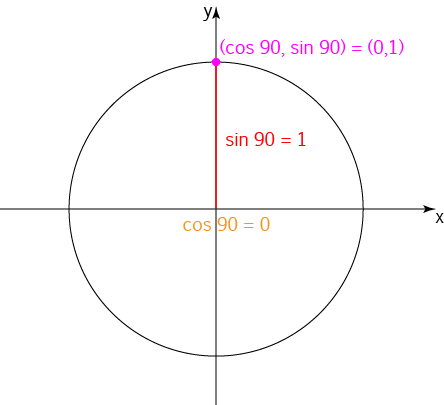If the angle $\theta$ that the line from $(0,0)$ to $(x,y)$ makes with the positive $x$-axis is $90^\circ$, then the $x$-coordinate of point $(x,y)$ is equal to $\cos 90 = 0$, and the $y$-coordinate is equal to $\sin 90 = 1$. The tangent of $90^\circ$ is undefined. So, the point has coordinates $(0,1)$.

Note: We know that the coordinates of different points around the unit circle will have positive $x$-coordinates, or negative $x$-coordinates, and similarly for their $y$-coordinates. This lines up nicely with the sine and cosine values of different angles having positive and negative values. We'll talk more about this later.

## Pythagoras' Theorem, The Unit Circle and Trigonometric Identities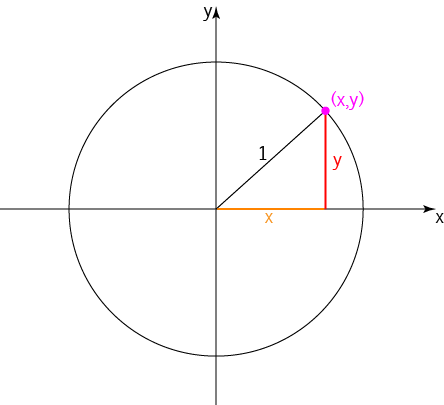The equation of the unit circle centred at the origin is $x^2 + y^2 = 1$. This corresponds to Pythagoras' theorem (the square on the hypotenuse of a right-angled triangle is equal to the sum of the squares on the other two sides) for a right-angled triangle with side lengths $x$ and $y$, and hypotenuse $1$:

\begin{align*} x^2 + y^2 &= 1^2\\ x^2 + y^2 &= 1 \end{align*}
We also know that $x = \cos\theta$ and $y = \sin \theta$, so we can use this relationship to establish the trigonometric identity:
\begin{align*} \cos^2 \theta + \sin^2 \theta &= 1 \end{align*}
This identity (equation that is always true, regardless of the value of $\theta$) is sometimes called the Pythagorean Trigonometric Identity. If you've never seen it before, it's a really good idea to memorise it. It's probably one of the most important items in your mathematical toolI kit: I've lost count of the number of times I've used it.

## Exact Angle Ratios

This is a slight digression to talk about the trig ratios for some important angles. My American students have been told to just memorise the ratios of the sides of these triangles. I think we're better off knowing how to work them out. So, we'll do just that in this section. I have never memorised these ratios, but I can very quickly work them out.

Don't worry, I'm not really going off on a tangent (apologies, I couldn't resist) - we'll need these ratios very soon.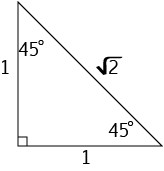The first one is the simplest to remember. Draw yourself an isosceles, right-angled triangle of side length one. By Pythagoras' theorem, the square of the hypotenuse is $1^2 + 1^2 = 2$, so the hypotenuse has length $\sqrt{2}$.

The triangle is isosceles, so its base angles are equal. As the other angle is $90^\circ$, they are each equal to $45^\circ$.

Now we can find our trig ratios (you might remember the mnemonic sohcahtoa):

• $\sin 45^\circ = \dfrac{\text{opposite}}{\text{hypotenuse}} = \dfrac{1}{\sqrt{2}}$
• $\cos 45^\circ = \dfrac{\text{adjacent}}{\text{hypotenuse}} = \dfrac{1}{\sqrt{2}}$
• $\tan 45^\circ = \dfrac{\text{opposite}}{\text{adjacent}} = \dfrac{\sqrt{2}}{\sqrt{2}} = 1$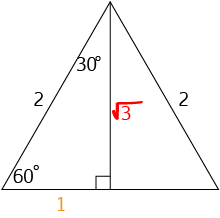Now draw yourself an equilateral triangle of side length two. Chop it in half using the perpendicular bisector of the base. This will also bisect the top angle of the triangle and form a right-angled triangle with hypotenuse $2$ and one of its side-lengths equal to $1$. We can find the length of the third side using Pythagoras' theorem. If it has length $a$, then by Pythagoras' theorem, the square of the hypotenuse is $1^2 + a^2 = 2^2$, so $a^2 = 3$ and $a = \sqrt{3}$. The angles of the right-angled triangle are $60^\circ$ (all angles of an equilateral triangle are equal to $60^\circ$) and $30^\circ$ (we bisected the other angle).

Now we can find our trig ratios (you might remember the mnemonic sohcahtoa):

• $\sin 30^\circ = \dfrac{\text{opposite}}{\text{hypotenuse}} = \dfrac{1}{2}$
• $\cos 30^\circ = \dfrac{\text{adjacent}}{\text{hypotenuse}} = \dfrac{\sqrt{3}}{2}$
• $\tan 30^\circ = \dfrac{\text{opposite}}{\text{adjacent}} = \dfrac{1}{\sqrt{3}}$
• $\sin 60^\circ = \dfrac{\text{opposite}}{\text{hypotenuse}} = \dfrac{\sqrt{3}}{2}$
• $\cos 60^\circ = \dfrac{\text{adjacent}}{\text{hypotenuse}} = \dfrac{1}{2}$
• $\tan 60^\circ = \dfrac{\text{opposite}}{\text{adjacent}} = \dfrac{\sqrt{3}}{1} = \sqrt{3}$

These are the exact angle ratios that I use, but you can sometimes lose marks if you write them in this form. It is, perhaps better, to rationalise the denominators of these ratios. The table gives each of these ratios after the denominators have been rationalised.

Angle Sine Cosine Tangent
$0^\circ$ $0$ $1$ $0$
$30^\circ$ $\dfrac{1}{2}$ $\dfrac{\sqrt{3}}{2}$ $\dfrac{\sqrt{3}}{3}$
$45^\circ$ $\dfrac{\sqrt{2}}{2}$ $\dfrac{\sqrt{2}}{2}$ $1$
$60^\circ$ $\dfrac{\sqrt{3}}{2}$ $\dfrac{1}{2}$ $\sqrt{3}$
$90^\circ$ $1$ $0$ undefined

## Plotting the Exact Coordinates On the Unit Circle

### The $x$-coordinates and Cosine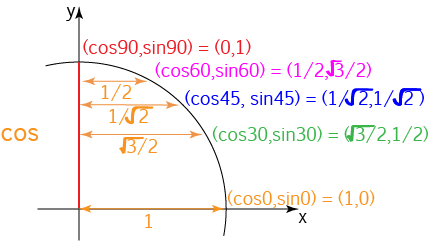As we move around the unit circle from the point $(1,0)$ in an anticlockwise direction, the size of the angle $\theta$ that a line joining our point to the origin makes with the positive $x$-axis increases. Our $x$-coordinate gets smaller as more of the length of the radius is taken up by moving upwards. So, the cosine of $\theta$ gets smaller as we move towards $90^\circ$, where it is equal to zero. This means that $\cos 30^\circ > \cos 45^\circ > \cos 60^\circ$, and so, if we don't remember the values of $\cos 30^\circ$ and $\cos 60^\circ$, we can get around the problem by remembering that $\cos 30^\circ$ is bigger, so it must be the one that's equal to $\dfrac{\sqrt{3}}{2}$, and $\cos 60^\circ$ is smaller, so it must be the one equal to $\dfrac{1}{2}$.

### The $x$-coordinates and Cosine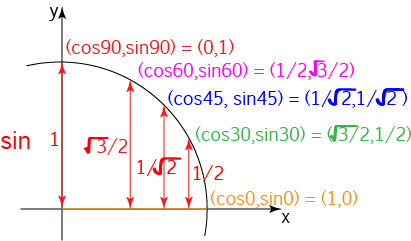As we move around the unit circle from the point $(1,0)$ in an anticlockwise direction, the size of the angle $\theta$ that a line joining our point to the origin makes with the positive $x$-axis increases. Our $y$-coordinate gets larger as the angle gets bigger. So, the sine of $\theta$ gets larger as we move towards $90^\circ$, where it is equal to 1. This means that $\sin 30^\circ ## Filling in the Rest of the Circle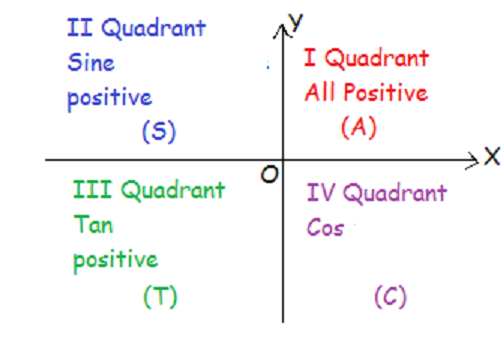This is one of the really cool things about this approach to trigonometry. Usually we have to remember that the \(xy$-plane is divided up into four quadrants. In the first quadrant sine, cosine and tangent are all positive. In the second quadrant sine is positive, and the others are negative. In the third quadrant, only tangent is positive, and in the fourth quadrant only cosine is positive. You've probably used a mnemonic like "All Stations to Central", or "All Seniors Turn Crazy" or "All Students Take Calculus" to remember this. If you haven't, you have a few options for remembering it now!

In the unit circle approach, we just use the properties of coordinates in the $xy$-plane, remembering that the $x$-coordinate corresponds to cosine, and the $y$-coordinate corresponds to sine. So, in the first quadrant, both coordinates are positive, so sine and cosine must both be positive. This means that tangent is also positive. In the second quadrant, the $x$-coordinate is negative, so cosine is negative, and the $y$-coordinate is positive, so sine is positive. This means that tangent negative. In the third quadrant, both $x$ and $y$ are negative. This means that both sine and cosine are negative, but tangent (being their ratio) is positive. Finally, in the fourth quadrant, $x$ is positive and $y$ is negative. So, cosine is positive, but both sine and tangent are negative.

The way the two approaches line up is nice, isn't it?

We mustn't forget that mathematicians really prefer to use radians. Remember the conversion: $180^\circ = \pi \text{ radians}$. We can label the angles in our unit circle using radians as well. The unit circle, with all the exact values filled in looks like this: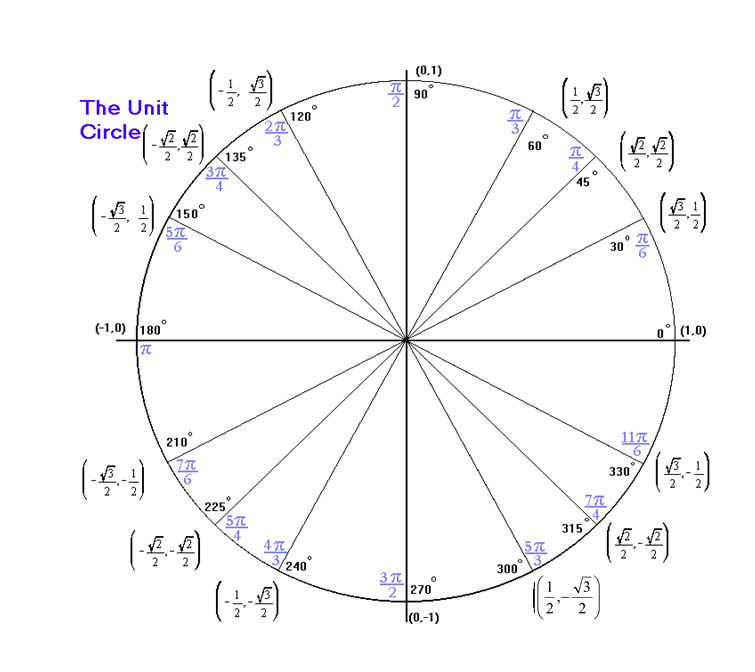Let's finish by using the unit circle to find the sines and cosines of a few angles.

### Example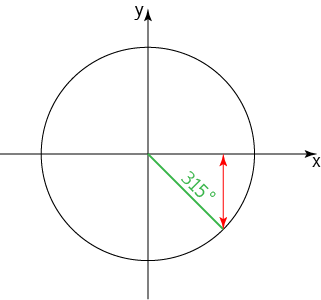What is the sine of $315^\circ$?

$315^\circ$ is equal to $360^\circ - 45^\circ$, but it is in the fourth quadrant, so its sine is negative. Therefore, $\sin 315^\circ = - \sin 45^\circ = - \dfrac{\sqrt{2}}{2}$.

### Example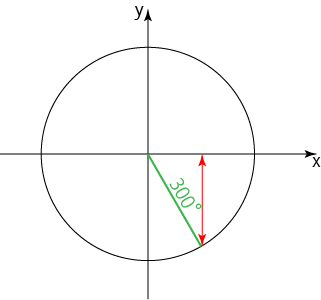What is the sine of $300^\circ$?

$300^\circ$ is equal to $360^\circ - 60^\circ$. It is further around the circle, so its sine is the larger value, but it is in the fourth quadrant, so its sine is negative. Therefore, $\sin 300^\circ = - \sin 60^\circ = - \dfrac{\sqrt{3}}{2}$.

### Example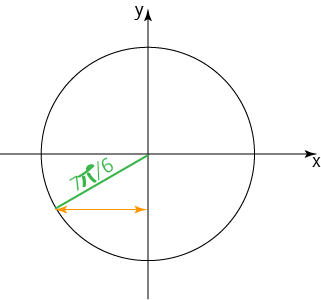What is the cosine of $\dfrac{7\pi}{6}$ radians?

$\dfrac{7\pi}{6}$ radians is equal to $\pi + \dfrac{\pi}{6}$ radians. Remember that $\dfrac{\pi}{6}$ is equal to $30^\circ$, so we have the larger distance from the $y$-axis. The angle is in the third quadrant, so its cosine is negative. Therefore, $\cos \dfrac{7\pi}{6} = - \cos \dfrac{\pi}{6} = - \dfrac{\sqrt{3}}{2}$.

### Description

coordinate geometry is a branch of geometry where the position of the points on the plane is defined with the help of an ordered pair of numbers also known as coordinates. In this tutorial series, you will learn about vast range of topics such as Cartesian Coordinates, Midpoint of a Line Segment etc

### Audience

year 10 or higher, several chapters suitable for Year 8+ students.

### Learning Objectives

Explore topics related to Coordinates Geometry

Author: Subject Coach
You must be logged in as Student to ask a Question.